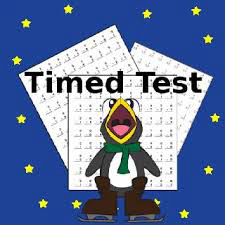• Save

How to improve Speed and accuracy in maths exams

Last month 40.7K ViewsThere is no substitute for hard work and intense practice. To improve speed of solving math problems one must work and practice very much.
However there are some points which are noteworthy in respect to all upcoming exams like CGL-14 tier-1 and tier 2 , IBPS, IB and all other exams.

1. Look carefully: Don’t just read the question only. look carefully at the options also before you begin solving the problem. Sometimes you might be lucky to find answer by putting value given in option back into question.
2. Take Random values: if possible, take random values and put those into question. Lots of tough questions can be solved by this way. For example:

Q-1   1/(1+2ˆa-b) +1/(1+2ˆb-a)  is a).  a-b b).  b-a c).   1 d).  0

[SSC-CGL TIER1 2013]

In this question if we put a=b=0 then we can easily find option c is the answer.

Q-2 If (1+sinα )(1+sinβ )(1+sinγ )=(1-sinα )(1-sinβ )(1-sinγ ), then each side is equal to
a. ±cosα cosβ cosγ
b. ±sinα sinβ sinγ
c. ±sinα cosβ cosγ
d. ±sinα sinβ cosγ [ SSC-CGL TIER1 2013]
Here put α =0,β =0,γ =0. This satisfies the equation so now check which option gives value 1 by putting values α =0,β =0,γ =0. clearly the answer is a.
Standard method to solve this question is following-
let (1+sinα )(1+sinβ )(1+sinγ )=k …. (1)
and (1-sinα )(1-sinβ )(1-sinγ )=k ….. (2)
now (1)×(2) gives
(1²-sinα ²)(1²-sinβ ²)(1²-sinγ ²)=k²
so k= ±cosα cosβ cosγ is the answer.

In the two examples above we have seen that by taking random values we may find answer easily hence no need for lengthy calculations.

3. Estimate Answers: If values given in options are far apart then by estimation we may find answer.

4. Geometry: If a question involves a triangle then assume a equilateral triangle(if possible). In an equilateral triangle, altituide, median, angle bisector, perpendicular bisector of each base are same. Centro-id, incentre, orthocentre and circumcentre are also the same. In some cases assuming right angle triangle would be suitable. Thus the problem would become simple.

Memorize these Pythagoras triplets-

3,4,5

5,12,13

7,24,25

8,15,17

multiples of these triplets are also pythagorian triplets. In most of questions you will find these triplets. We can say a traingle is right angle if sides are pythagorian triplets.

5. Practice the weaker sections: One must study at home carefully and find similar things which can improve his performance.  Some students find problems of ‘Time and Work’ difficult while others find problem on triangles a bit difficult. Extra time should be given on your weak sections. Do a lot of questions. The more questions you solve the more smart you become!

6. Note down and remember formulas: It is a good habit to note down in a separate paper all important formulas, conversions, expressions, tricks you required while solving questions. And stick this paper to wall in front of your study table.  This way you will be able to memorize them quickly.  Before going to exam have a look on those papers, you won’t forget these in Exam.

7. Don’t memorize Tricks: Don’t memorize too many tricks as given in many books. It just creates more confusion in exam time. Memorize only those tricks which you yourself found helpful and important at time of preparation, none else.

8. practice using using rough space:At home we are in habit of using rough space lavishly. Be prepared! In exam you wont find this much space. Space is limited in question paper.  If you have seen the real paper of Maths SSC-CGL TIER- 2 2012  you would find very limited space. Many students were annoyed and expressed their displeasure over this. Hope SSC gives enough space this year, yet one should adjust himself to limited space and overwriting.

9.  read the questions carefully:

In exam read the questions carefully and look the question in Hindi language also if required.

10. Don’t get anxious:  When the bell for last 30 minutes rings many students get anxious as their performance was below their expectations and try to solve questions quickly. Sorry this doesn’t help! Be calm and focus on questions and pick those which look easy. Your performance might be low because of a very tough paper and cut-off would be low.

11. Concentration: Concentration is the best instrument to enhance your speed and accuracy. Put your heart and soul into the question.  If the invigilators are chatting unnecessarily ask them to stop talking.

Friends comment below to give feedback about article and share it to your friends. If your are preparing for SSC CGL then read: SSC CGL Tier-2 paper composition and cut off analysis and SSC CGL Tier-2 Tips and tricks.

We wish you the best for exam!